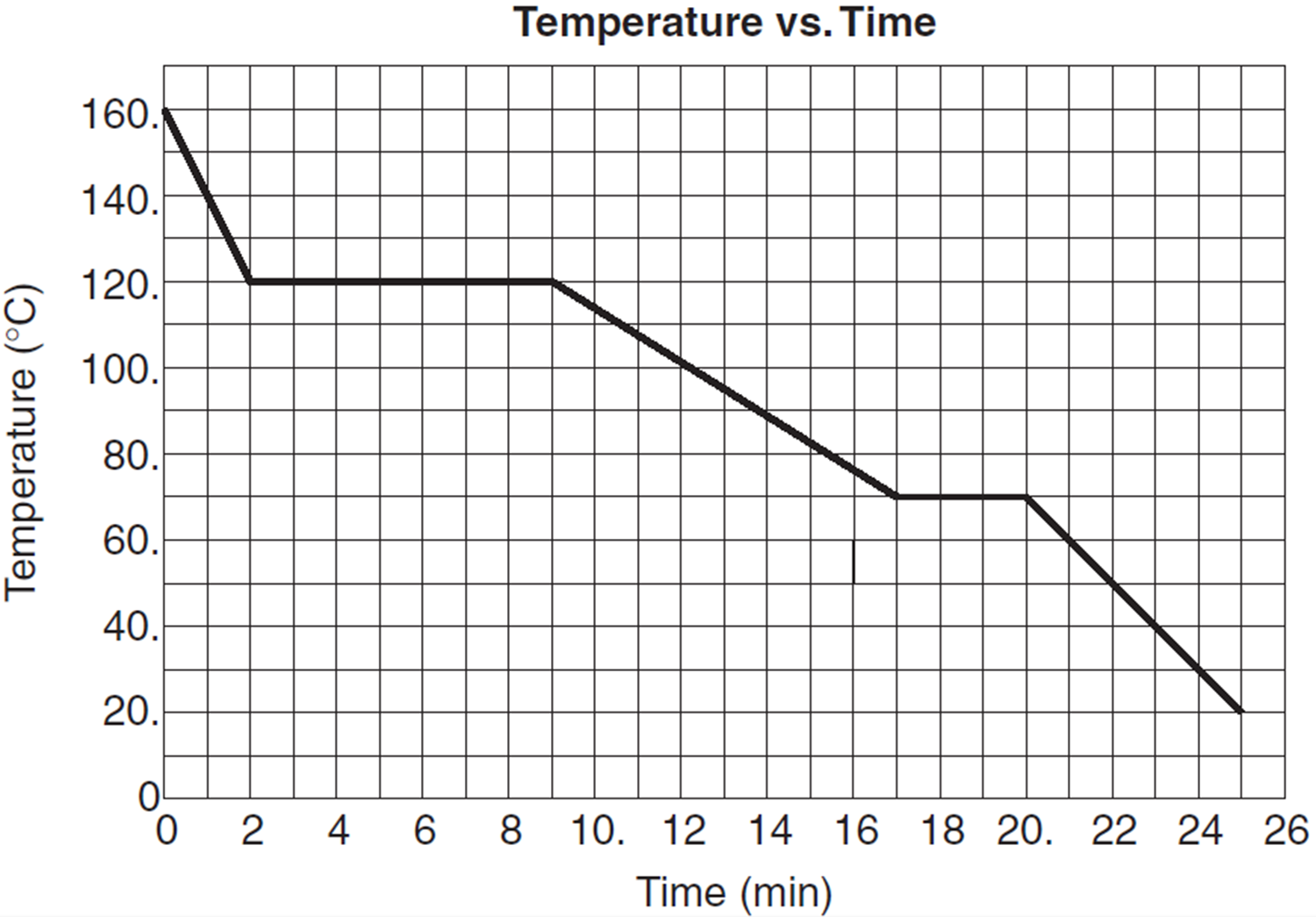# Phase Changes

As you know, matter can exist in different states. These states include solids, liquids, gases, and plasmas. You’re probably familiar with solids, liquids, and gases already. Plasmas are energetic gases that have been ionized so that they can conduct electricity (examples include stars, lightning, neon signs, etc.)

When matter changes state, its internal energy changes, so the kinetic energy of its constituent particles changes. As it is changing from one state to another, the change in energy is reflected in the bonds between the particles, and therefore the temperature of the object doesn’t change. Once the state change is complete, however, changes in energy are again observed in the form of changes in temperature.The energy required to change a specific material’s state is known as the material’s latent heat of transformation (L). When an object transitions from the solid to liquid phase, you use the latent heat of fusion (Lf). When an object transitions from the liquid to the gaseous phase, you use the latent heat of vaporization (Lv). You can calculate the energy required for a material to change phases using the following formula, where Q is the heat added, m is the object’s mass, and L is the material’s specific latent heat of transformation.Question: The graph below represents a cooling curve for 10 kilograms of a substance as it cools from a vapor at 160°C to a solid at 20°C. Energy is removed from the sample at a constant rate.While the substance is cooling during the liquid phase, the average kinetic energy of the molecules of the substance

1. decreases
2. increases
3. remains the same

Answer: (1) decreases since the temperature decreases, and average kinetic energy is related to temperature.

Question: Based on the graph of the previous problem, what is the melting point of the substance?

Question: How much heat must be added to a 10 kg lead bar to change the bar from a solid to a liquid at 327°C?

Answer:Question: How much heat must be added to 1 kg of water to change it from a 50°C to 100°C steam at standard pressure?

Answer: To solve this problem, you must find both the amount of heat required to change the temperature of the water, as well as the amount of heat required to change the state of the water.Question: The graph below shows temperature vs. time for one kilogram of an unknown material as heat is added at a constant rate.During interval xy, the material experiences

1. a decrease in internal energy and a phase change
2. an increase in internal energy and a phase change
3. no change in internal energy and a phase change
4. no change in internal energy and no phase change

Answer: (2) an increase in internal energy and a phase change.